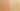# PlusConcatenatesNumbersDRAFT

Misconception:

Given a string and a number, one can use the plus operator to concatenate the two into a string. For example `"1 + 2 = " + 3` will evaluate to `"1 + 2 = 3"`.Incorrect

The plus operator can concatenate strings and numbers

Correct

## CorrectionHere is what's right.

In Python, the `+` operator is overloaded. The arithmetic plus operator adds two numbers (e.g., `1 + 2`). The concatenation operator concatenates two sequences (e.g., the two strings `"Hello" + "World"`).

However, Python does not have a plus operator that concatenates a string and a number. To concatenate a string and a number, one can convert the number to a string using `str()`, and then concatenate the two strings:

``````text = "The answer is "
number = 42
print(text + str(number))``````

The resulting expression tree looks as follows. While the variable `number` contains a number, the `str()` function converts that into the corresponding string. The plus operator then can simply concatenate two strings.

## ValueHow can you build on this misconception?

In other languages, for example in Java, the plus operator can indeed concatenate strings and numbers.

Python